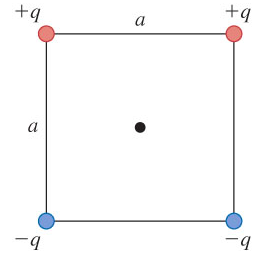# Problem: A point charge is placed at each comer of a square with side length a. The charges all have the same magnitude q. Two of the charges are positive and two are negative, as shown in the following figure.Part AWhat is the direction of the net electric field at the center of the square?a) leftward directionb) upward directionc) rightward directiond) downward directionPart   BWhat is the magnitude of the net electric field at the center of the square due to the four charges in terms of q and a?Express your answer in terms of the variables q, a, and appropriate constants.

###### FREE Expert Solution

In this problem, we are required to calculate and add electric fields.

Electric field:

$\overline{){\mathbf{E}}{\mathbf{=}}\frac{\mathbf{k}\mathbf{q}}{{\mathbf{r}}^{\mathbf{2}}}}$

95% (163 ratings)###### Problem Details

A point charge is placed at each comer of a square with side length a. The charges all have the same magnitude q. Two of the charges are positive and two are negative, as shown in the following figure.Part A

What is the direction of the net electric field at the center of the square?

a) leftward direction

b) upward direction

c) rightward direction

d) downward direction

Part   B

What is the magnitude of the net electric field at the center of the square due to the four charges in terms of q and a?

Express your answer in terms of the variables q, a, and appropriate constants.